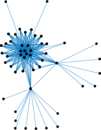# European Union domain

This is the hyperlink network of the .eu domain, for the European Union.

 Code `EU` Internal name `dimacs10-eu-2005` Name European Union domain Data source https://www.cc.gatech.edu/dimacs10/archive/clustering.shtml AvailabilityDataset is available for download Consistency checkDataset passed all tests Category Hyperlink network Dataset timestamp 2005 Node meaning Page Edge meaning Hyperlink Network format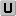Unipartite, undirected Edge typeUnweighted, no multiple edges LoopsDoes not contain loops Snapshot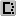Is a snapshot and likely to not contain all data Orientation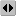Is not directed, but the underlying data is Multiplicity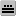Does not have multiple edges, but the underlying data has

## Statistics

 Size n = 862,664 Volume m = 16,138,468 Loop count l = 0 Wedge count s = 41,592,855,049 Claw count z = 399,568,418,872,993 Cross count x = 4,194,998,147,314,370,048 Triangle count t = 202,170,577 Square count q = 262,745,367,033 Maximum degree dmax = 68,963 Average degree d = 37.415 4 Fill p = 4.337 20 × 10−5 Size of LCC N = 862,664 Diameter δ = 21 50-Percentile effective diameter δ0.5 = 4.204 69 90-Percentile effective diameter δ0.9 = 6.242 29 Median distance δM = 5 Mean distance δm = 4.911 12 Gini coefficient G = 0.652 140 Balanced inequality ratio P = 0.261 425 Relative edge distribution entropy Her = 0.898 321 Power law exponent γ = 1.366 01 Tail power law exponent γt = 2.611 00 Clustering coefficient c = 0.014 582 1 Spectral norm α = 738.130 Non-bipartivity bA = 0.018 208 5 Normalized non-bipartivity bN = 8.978 75 × 10−5 Algebraic non-bipartivity χ = 0.000 180 101 Spectral bipartite frustration bK = 1.203 39 × 10−6

## Plots

### Degree distribution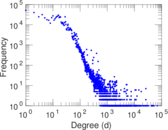### Cumulative degree distribution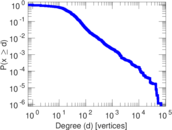### Spectral graph drawing based on the normalized adjacency matrix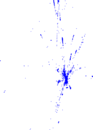### Zipf plot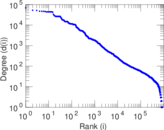### Hop distribution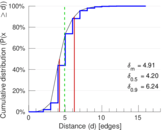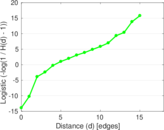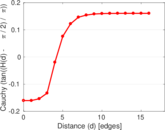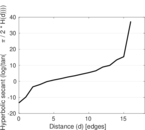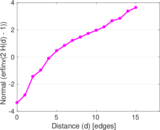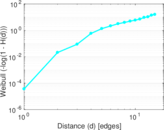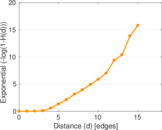### Clustering coefficient distribution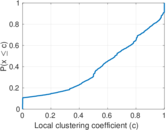### SynGraphy